#### AntiCommutator

##### Description

AntiCommutator[x, y] = c defines the anti-commutator of the non commuting objects x and y.

##### Examples

This declares a and b as noncommutative variables.

``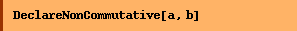``
``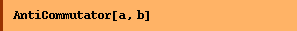``
`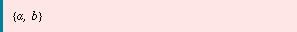`
``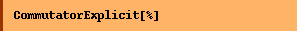``
`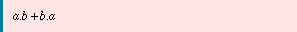`
``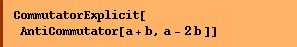``
`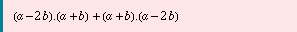`
``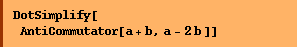``
`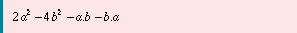`
``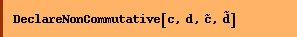``

Defining {c,d} = z results in replacements of c.d by z-d.c.

``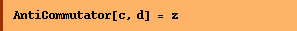``
`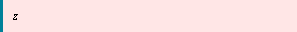`
``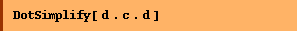``
`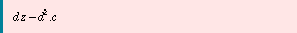`
``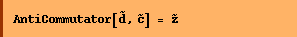``
`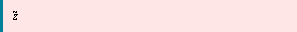`
``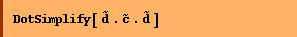``
`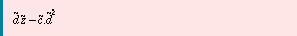`
``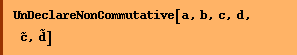``
``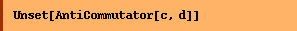``
``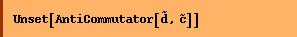``

Converted from the Mathematica notebook AntiCommutator.nb